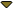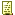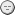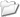You are not logged in.   Log In  |  Register PG Music Home » Forums » User-to-User » UserTracks (and other add-ons) » Free Philharmonia Orchestra Instruments/Percussion Samples New User    Forum List     Latest TopicsCalendar     Forum Rules     FAQ
 You need to be logged in to post
 Options#435242 - 10/26/17 02:53 AM [UserTracks (and other add-ons)]Re: Free Philharmonia Orchestra Instruments/Percussion Samples Registered: 02/05/15 Posts: 7294 PipelineVeteran Registered: 02/05/15 Posts: 7294 Sorry I thought they were already sfz.Get http://vis.versilstudios.net/sfzconverter.htmlYou can create different instruments with the different articulations or you can create keyswitches see Here, so sort the different articulation of each instrument into separate folders first. you need to save these 2 text files to FolderToSFZ_v060\NotationDictionaries as they have "s" for sharp instead of "#" in the filename.Copy and Save As C3=60 s=sharp.txtClick to reveal.. Code:```Notation=KeyNumber C-2=0 Cs-2=1 Db-2=1 D-2=2 Ds-2=3 Eb-2=3 E-2=4 F-2=5 Fs-2=6 Gb-2=6 G-2=7 Gs-2=8 Ab-2=8 A-2=9 As-2=10 Bb-2=10 B-2=11 C-1=12 Cs-1=13 Db-1=13 D-1=14 Ds-1=15 Eb-1=15 E-1=16 F-1=17 Fs-1=18 Gb-1=18 G-1=19 Gs-1=20 Ab-1=20 A-1=21 As-1=22 Bb-1=22 B-1=23 C0=24 Cs0=25 Db0=25 D0=26 Ds0=27 Eb0=27 E0=28 F0=29 Fs0=30 Gb0=30 G0=31 Gs0=32 Ab0=32 A0=33 As0=34 Bb0=34 B0=35 C1=36 Cs1=37 Db1=37 D1=38 Ds1=39 Eb1=39 E1=40 F1=41 Fs1=42 Gb1=42 G1=43 Gs1=44 Ab1=44 A1=45 As1=46 Bb1=46 B1=47 C2=48 Cs2=49 Db2=49 D2=50 Ds2=51 Eb2=51 E2=52 F2=53 Fs2=54 Gb2=54 G2=55 Gs2=56 Ab2=56 A2=57 As2=58 Bb2=58 B2=59 C3=60 Cs3=61 Db3=61 D3=62 Ds3=63 Eb3=63 E3=64 F3=65 Fs3=66 Gb3=66 G3=67 Gs3=68 Ab3=68 A3=69 As3=70 Bb3=70 B3=71 C4=72 Cs4=73 Db4=73 D4=74 Ds4=75 Eb4=75 E4=76 F4=77 Fs4=78 Gb4=78 G4=79 Gs4=80 Ab4=80 A4=81 As4=82 Bb4=82 B4=83 C5=84 Cs5=85 Db5=85 D5=86 Ds5=87 Eb5=87 E5=88 F5=89 Fs5=90 Gb5=90 G5=91 Gs5=92 Ab5=92 A5=93 As5=94 Bb5=94 B5=95 C6=96 Cs6=97 Db6=97 D6=98 Ds6=99 Eb6=99 E6=100 F6=101 Fs6=102 Gb6=102 G6=103 Gs6=104 Ab6=104 A6=105 As6=106 Bb6=106 B6=107 C7=108 Cs7=109 Db7=109 D7=110 Ds7=111 Eb7=111 E7=112 F7=113 Fs7=114 Gb7=114 G7=115 Gs7=116 Ab7=116 A7=117 As7=118 Bb7=118 B7=119 C8=120 Cs8=121 Db8=121 D8=122 Ds8=123 Eb8=123 E8=124 F8=125 Fs8=126 Gb8=126 G8=127 ```Copy and Save As C4=60 s=sharp.txtClick to reveal.. Code:```Notation=KeyNumber C-1=0 Cs-1=1 Db-1=1 D-1=2 Ds-1=3 Eb-1=3 E-1=4 F-1=5 Fs-1=6 Gb-1=6 G-1=7 Gs-1=8 Ab-1=8 A-1=9 As-1=10 Bb-1=10 B-1=11 C0=12 Cs0=13 Db0=13 D0=14 Ds0=15 Eb0=15 E0=16 F0=17 Fs0=18 Gb0=18 G0=19 Gs0=20 Ab0=20 A0=21 As0=22 Bb0=22 B0=23 C1=24 Cs1=25 Db1=25 D1=26 Ds1=27 Eb1=27 E1=28 F1=29 Fs1=30 Gb1=30 G1=31 Gs1=32 Ab1=32 A1=33 As1=34 Bb1=34 B1=35 C2=36 Cs2=37 Db2=37 D2=38 Ds2=39 Eb2=39 E2=40 F2=41 Fs2=42 Gb2=42 G2=43 Gs2=44 Ab2=44 A2=45 As2=46 Bb2=46 B2=47 C3=48 Cs3=49 Db3=49 D3=50 Ds3=51 Eb3=51 E3=52 F3=53 Fs3=54 Gb3=54 G3=55 Gs3=56 Ab3=56 A3=57 As3=58 Bb3=58 B3=59 C4=60 Cs4=61 Db4=61 D4=62 Ds4=63 Eb4=63 E4=64 F4=65 Fs4=66 Gb4=66 G4=67 Gs4=68 Ab4=68 A4=69 As4=70 Bb4=70 B4=71 C5=72 Cs5=73 Db5=73 D5=74 Ds5=75 Eb5=75 E5=76 F5=77 Fs5=78 Gb5=78 G5=79 Gs5=80 Ab5=80 A5=81 As5=82 Bb5=82 B5=83 C6=84 Cs6=85 Db6=85 D6=86 Ds6=87 Eb6=87 E6=88 F6=89 Fs6=90 Gb6=90 G6=91 Gs6=92 Ab6=92 A6=93 As6=94 Bb6=94 B6=95 C7=96 Cs7=97 Db7=97 D7=98 Ds7=99 Eb7=99 E7=100 F7=101 Fs7=102 Gb7=102 G7=103 Gs7=104 Ab7=104 A7=105 As7=106 Bb7=106 B7=107 C8=108 Cs8=109 Db8=109 D8=110 Ds8=111 Eb8=111 E8=112 F8=113 Fs8=114 Gb8=114 G8=115 Gs8=116 Ab8=116 A8=117 As8=118 Bb8=118 B8=119 C9=120 Cs9=121 Db9=121 D9=122 Ds9=123 Eb9=123 E9=124 F9=125 Fs9=126 Gb9=126 G9=127 ``` Attachments Post attachments only available when logged in _________________________ ReaTrak Biab & Plugin in ReaperFree Biab Chord Picker Tool Top

 Entire topic Subject Posted by PostedFree Philharmonia Orchestra Instruments/Percussion Samples Pipeline 08/17/17 11:34 PMRe: Free Philharmonia Orchestra Instruments/Percussion Samples VideoTrack 08/18/17 05:22 AMRe: Free Philharmonia Orchestra Instruments/Percussion Samples 90 dB 08/18/17 10:07 AMRe: Free Philharmonia Orchestra Instruments/Percussion Samples Hugh2 08/18/17 04:32 PMRe: Free Philharmonia Orchestra Instruments/Percussion Samples Al-David 10/24/17 05:50 PMRe: Free Philharmonia Orchestra Instruments/Percussion Samples Pipeline 10/24/17 08:38 PMRe: Free Philharmonia Orchestra Instruments/Percussion Samples Bernard 10/26/17 01:14 AMRe: Free Philharmonia Orchestra Instruments/Percussion Samples Pipeline 10/26/17 02:53 AMRe: Free Philharmonia Orchestra Instruments/Percussion Samples David Snyder 10/29/17 06:24 PM